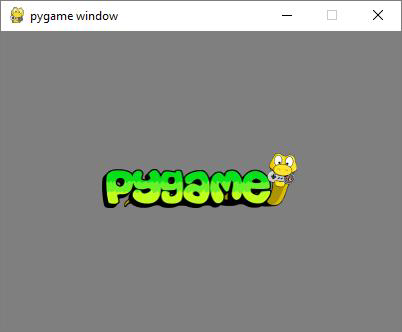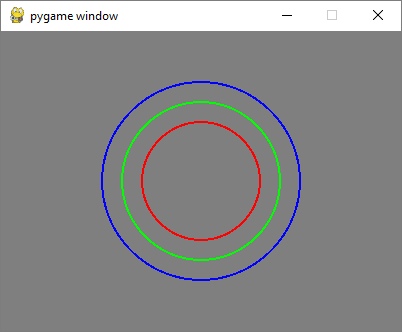The pygame.image module contains functions for loading and saving images from file or file like object. An image is loaded as a Surface object which eventually is rendered on Pygame display window.

First we obtain a Surface object by load() function.

img = pygame.image.load('pygame.png')


Next we obtain a rect object out of this Surface and then use Surface.blit() function to render the image −

rect = img.get_rect()
rect.center = 200, 150
screen.blit(img, rect)


## Example

The complete program for displaying Pygame logo on the display window is as follows −

import pygame

pygame.init()
screen = pygame.display.set_mode((400, 300))
done = False
bg = (127,127,127)
while not done:
for event in pygame.event.get():
screen.fill(bg)
rect = img.get_rect()
rect.center = 200, 150
screen.blit(img, rect)
if event.type == pygame.QUIT:
done = True
pygame.display.update()


## Output

The output for the above code is as follows −The blit() function can take an optional special-flags parameter with one of the following values −

BLEND_RGBA_ADD
BLEND_RGBA_SUB
BLEND_RGBA_MULT
BLEND_RGBA_MIN
BLEND_RGBA_MAX
BLEND_RGB_SUB
BLEND_RGB_MULT
BLEND_RGB_MIN
BLEND_RGB_MAX


The pygame.Surface module also has a convert() function which optimizes the image format and makes drawing faster.

The pygame.image module has a save() function that saves contents of Surface object to an image file. Pygame supports the following image formats −

JPG

PNG

GIF (non-animated)

BMP

PCX

TGA (uncompressed)

TIF

LBM (and PBM)

PBM (and PGM, PPM)

XPM

BMP

TGA

PNG

JPEG

## Example

Following program draws three circles on the display surface and save it as a circles.png file using image.save() function.

import pygame

pygame.init()
screen = pygame.display.set_mode((400, 300))
done = False
white=(255,255,255)
red = (255,0,0)
green = (0,255,0)
blue = (0,0,255)

bg = (127,127,127)
while not done:
for event in pygame.event.get():
screen.fill(bg)
if event.type == pygame.QUIT:
done = True
pygame.draw.circle(screen, red, (200,150), 60,2)
pygame.draw.circle(screen, green, (200,150), 80,2)
pygame.draw.circle(screen, blue, (200,150), 100,2)
pygame.display.update()
pygame.image.save(screen, "circles.png")


## OutputThe circles.png should be created in the current working folder.